JEE  >  Irodov Solutions: Mechanical Oscillations- 7

# Irodov Solutions: Mechanical Oscillations- 7 - I. E. Irodov Solutions for Physics Class 11 & Class 12 - JEE

Q.81. A conductor in the shape of a square frame with side a suspended by an elastic thread is located in a uniform horizontal magnetic field with induction B. In equilibrium the plane of the frame is parallel to the vector B (Fig. 4.25). Having been displaced from the equilibrium position, the frame performs small oscillations about a vertical axis passing through its centre. The moment of inertia of the frame relative to that axis is equal to I, its electric resistance is R. Neglecting the inductance of the frame, find the time interval after which the amplitude of the frame's deviation angle decreases e-fold.

Ans. If φ = angle of deviation of the frame from its normal position, then an e.m.f.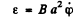is induced in the frame in the displaced position and a current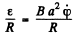flows in it. A couple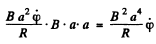then acts on the frame in addition to any elastic restoring couple c φ. We write the equation of the frame as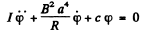Thus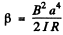where β is defined in the book.

Amplitude of oscillation die out according to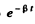so time required for the oscillations to decrease to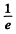of its value is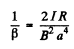Q.82. A bar of mass m = 0.50 kg lying on a horizontal plane with a friction coefficient k = 0.10 is attached to the wall by means of a horizontal non-deformed spring. The stiffness of the spring is equal to x = 2.45 N/cm, its mass is negligible. The bar was displaced so that the spring was stretched by x0 = 3.0 cm, and then released. Find:
(a) the period of oscillation of the bar;
(b) the total number of oscillations that the bar performs until it stops completely.

Ans. We shall denote the stiffness constant by k . Suppose the spring is stretched by x0 . The bar in then subject to two horizontal forces (1) restorin g force - k x and (2) friction kmg opposing motion. If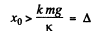the bar will come back.

(If x0 ≤ Δ , the bar will stay put) The equation of the bar when it is moving to the left is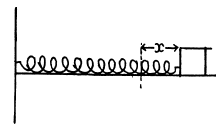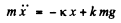This equation has the solution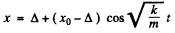where we have used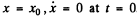This solution is only valid till the bar comes to rest. This happens at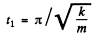and at that time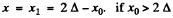the tendency of the rod will now be to move to the right . (if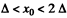the rod will stay put now) Now the equation for rightward motion becomes'*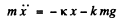(the friction force has reversed ).

We notice that the rod will move to the right only if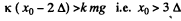In this case the solution is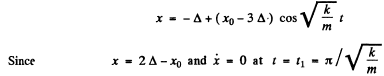The rod will next come to rest at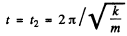and at that instant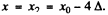. How ever the rod will stay put unless x0 > 5Δ. Thus

(a) time period of one full oscillation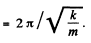(b) There is no oscillation if 0 < x0 < Δ

One half oscillation if Δ < x0 < 3Δ

2 half oscillation if 3Δ < x0< 5Δ etc.
We can say that the number of full oscillations is one half of the integer n

where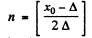where [ x ] = smallest non-negative integer greater than x.

Q.83. A ball of mass m can perform undamped harmonic oscillations about the point x = 0 with natural frequency ωo. At the moment t = 0, when the ball was in equilibrium, a force Fx = F cos ωt coinciding with the x axis was applied to it. Find the law of forced oscillation x (t) for that ball.

Ans. The equation of motion of the ball is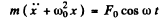.

This equation has the solution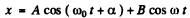where A and a are arbitrary and B is obtained by substitution in the above equation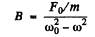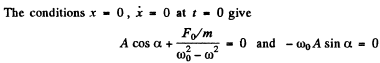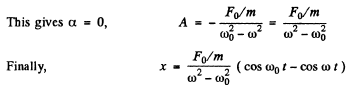Q.84. A particle of mass m can perform undamped harmonic oscillations due to an electric force with coefficient k. When the particle was in equilibrium, a permanent force F was applied to it for δ seconds. Find the oscillation amplitude that the particle acquired after the action of the force ceased. Draw the approximate plot x (t) of oscillations. Investigate possible cases.

Ans. We have to look for solutions of the equation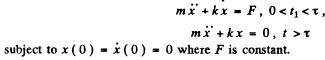The solution of this equation will be sought in the form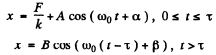A and α will be determined from the boundary condition at t = 0.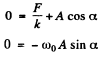Thus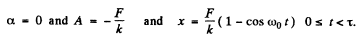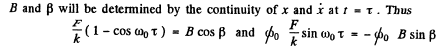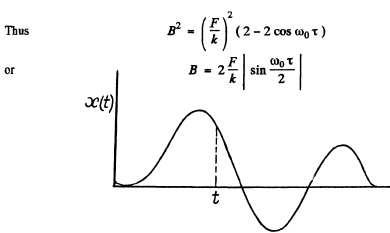Q.85. A ball of mass m, when suspended by a spring stretches the latter by Δl. Due to external vertical force varying according to a harmonic law with amplitude F0  the ball performs forced oscillations. The logarithmic damping decrement is equal to λ. Neglecting the mass of the spring, find the angular frequency of the external force at which the displacement amplitude of the ball is maximum. What is the magnitude of that amplitude?

Ans. Foi the spring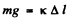where k is its stifiiess coefficient. Thus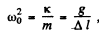The equation of motion of the ball is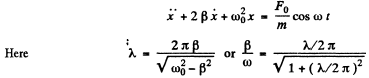To find the solution of the above equation we look for the solution of the auxiliary equation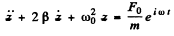Clearly we can take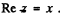Now we look for a particular integral for z of the form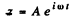Thus, substitution gives A and we get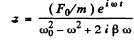so taking the real part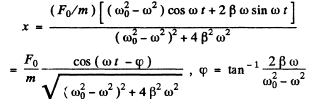The amplitude of this oscillation is maximum when the denominator is minimum. Tthis happens when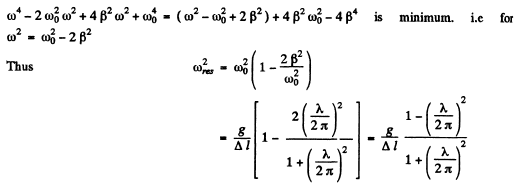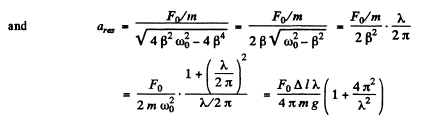Q.86. The forced harmonic oscillations have equal displacement amplitudes at frequencies ω1 = 400 s-1  and ω= 600s-1 . Find the resonance frequency at which the displacement amplitude is maximum.

Ans.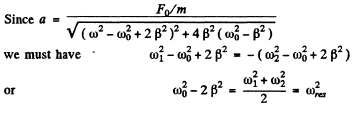Q.87. The velocity amplitude of a particle is equal to half the maximum value at the frequencies ω1  and ω2 of external harmonic force. Find:
(a) the frequency corresponding to the velocity resonance;
(b) the damping coefficient β and the damped oscillation frequency co of the particle.

Ans.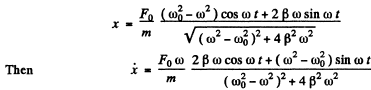Thus the velocity amplitude is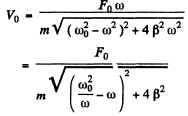This is maximum when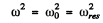and then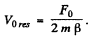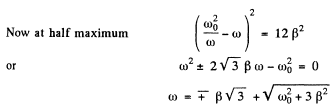where we have rejected a solution with - ve sign before there dical. Writing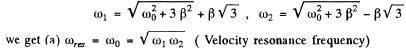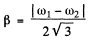and damped oscillation frequency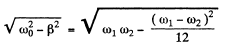Q.88. A certain resonance curve describes a mechanical oscillating system with logarithmic damping decrement λ = 1.60. For this curve find the ratio of the maximum displacement amplitude to the displacement amplitude at a very low frequency.

Ans. In general for displacement amplitude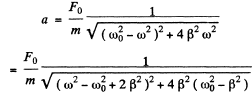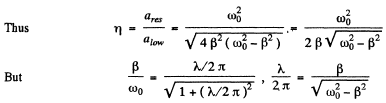Hence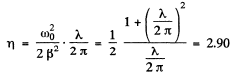Q.89. Due to the external vertical force Fx  = F cos ωt a body suspended by a spring performs forced steady-state oscillations according to the law x = a cos (ωt — φ). Find the work performed by the force F during one oscillation period.

Ans. The work done in one cycle is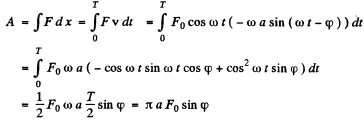Q.90. A ball of mass m = 50 g is suspended by a weightless spring with stiffness x = 20.0 N/m. Due to external vertical harmonic force with frequency ω = 25.0 s-1  the ball performs steady-state oscillations with amplitude a = 1.3 cm. In this case the displace- ment of the ball lags in phase behind the external force by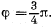Find: (a) the quality factor of the given oscillator; (b) the work performed by the external force during one oscillation period.

Ans. In the formula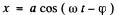we have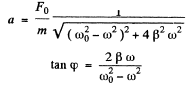Thus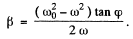Hence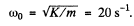and (a) the quality factor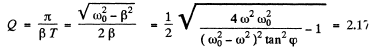(b) work done is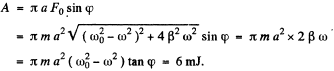Q.91. A ball of mass m suspended by a weightless spring can perform vertical oscillations with damping coefficient β. The natural oscillation frequency is equal to ω0. Due to the external vertical force varying as Fx = Fo , cos ωt the ball performs steady-state harmonic oscillations. Find:
(a) the mean power (P), developed by the force F, averaged over one oscillation period;
(b) the frequency ω of the force F at which (P) is maximum; what is (P)max equal to?

Ans. Here as usual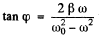where φ is the phase lag of the displacement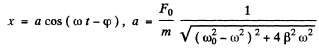(a) Mean power developed by the force over one oscillation period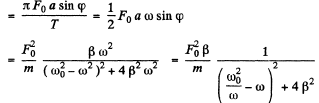(b) Mean power < P > is maximum when ω = ω0 (for the denominator is then minimuir Also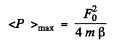Q.92. An external harmonic force F whose frequency can be varied, with amplitude maintained constant, acts in a vertical direction on a ball suspended by a weightless spring. The damping coefficient is times less than the natural oscillation frequency ωo of the ball. How much, in per cent, does the mean power (P) developed by the force F at the frequency of displacement resonance differ from the maximum mean power (P)max?  Averaging is performed over one oscillation period.

Ans. Given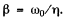Then from the previous problem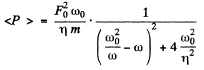At displacement resonance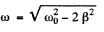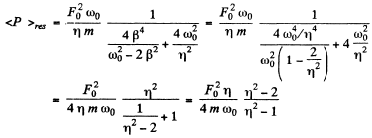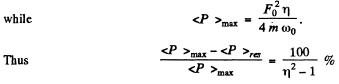Q.93. A uniform horizontal disc fixed at its centre to an elastic vertical rod performs forced torsional oscillations due to the moment of forces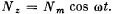The oscillations obey the law φ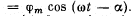Find: (a) the work performed by friction forces acting on the disc during one oscillation period; (b) the quality factor of the given oscillator if the moment of inertia of the disc relative to the axis is equal to I.

Ans. The equation of the disc is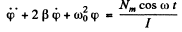Then as before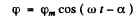where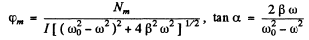(a) Work performed by frictional forces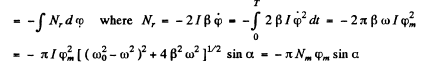(b) The quality factor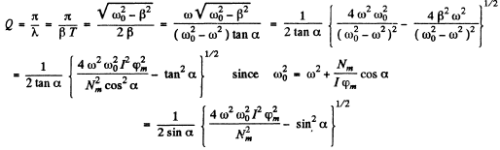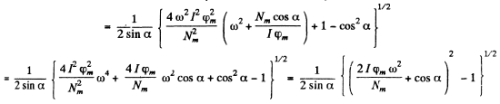The document Irodov Solutions: Mechanical Oscillations- 7 | I. E. Irodov Solutions for Physics Class 11 & Class 12 - JEE is a part of the JEE Course I. E. Irodov Solutions for Physics Class 11 & Class 12.
All you need of JEE at this link: JEE

106 docs

## I. E. Irodov Solutions for Physics Class 11 & Class 12

106 docsExplore Courses for JEE examSignup to see your scores go up within 7 days! Learn & Practice with 1000+ FREE Notes, Videos & Tests.
10M+ students study on EduRev
Track your progress, build streaks, highlight & save important lessons and more!
Related Searches

,

,

,

,

,

,

,

,

,

,

,

,

,

,

,

,

,

,

,

,

,

;